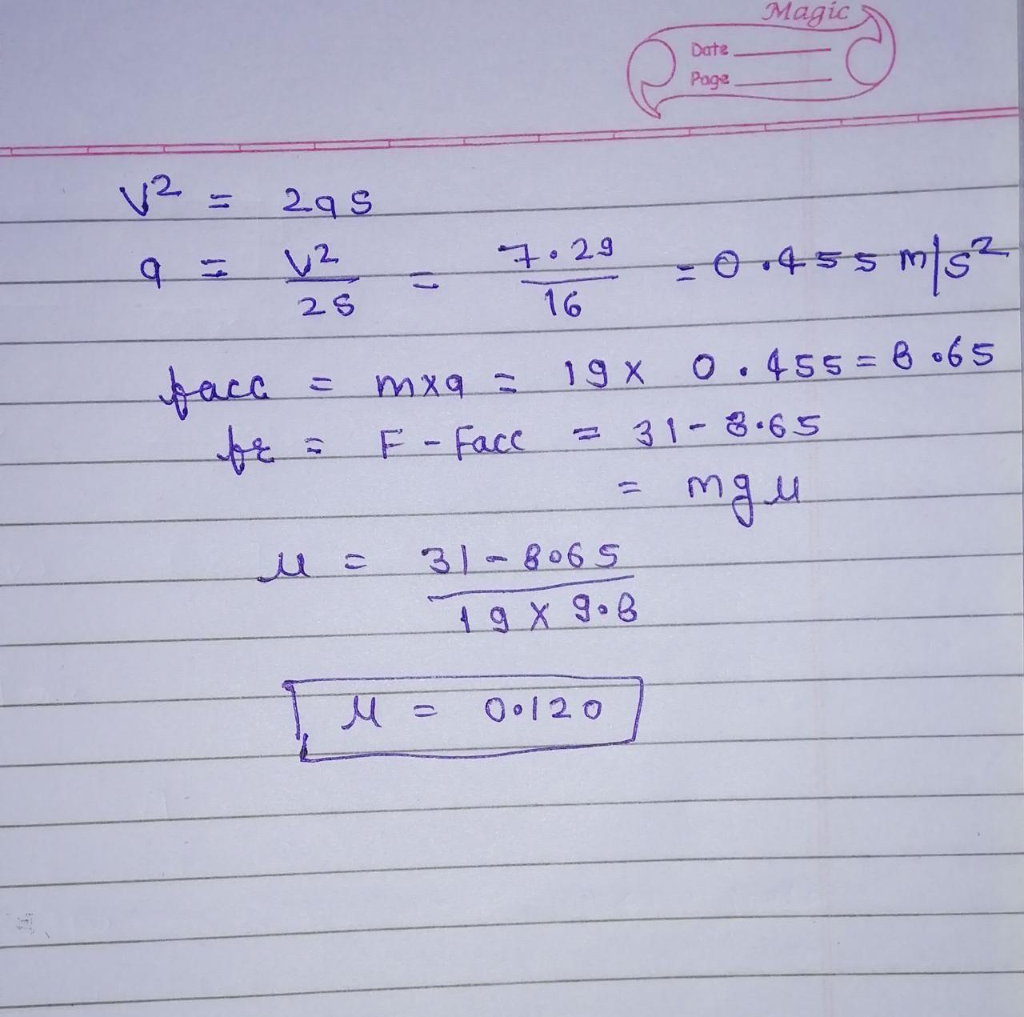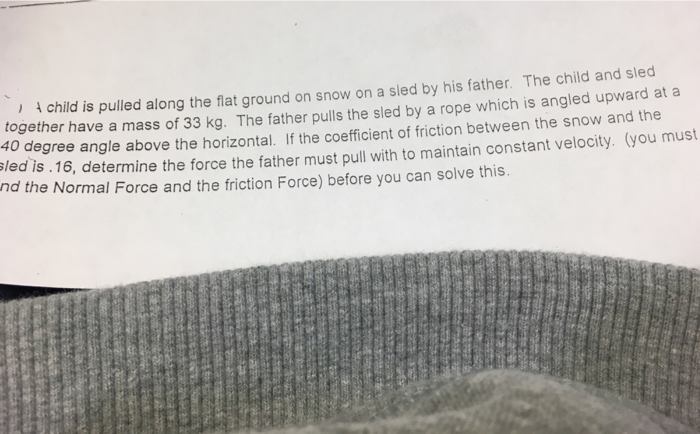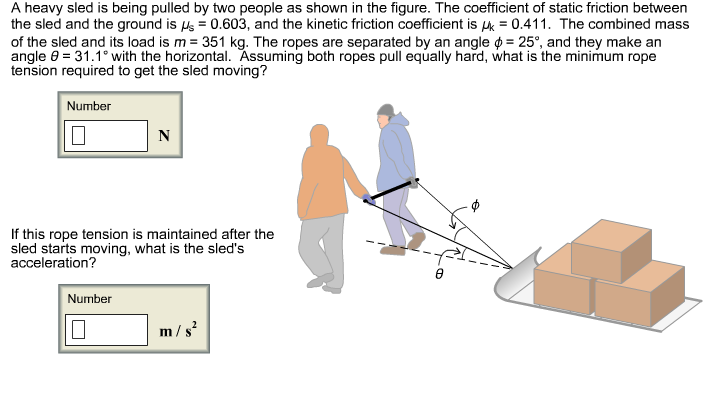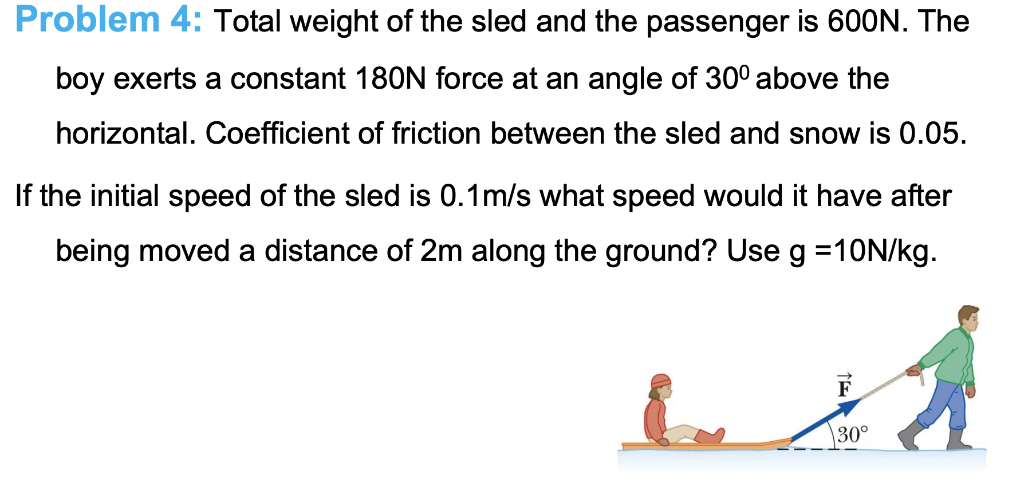Question

# A 19-kg sled is being pulled along the horizontal snow-covered ground by a horizontal force of...

A 19-kg sled is being pulled along the horizontal snow-covered ground by a horizontal force of 31 N. Starting from rest, the sled attains a speed of 2.7 m/s in 8.0 m. Find the coefficient of kinetic friction between the runners of the sled and the snow. Number Units#### Earn Coins

Coins can be redeemed for fabulous gifts.

Similar Homework Help Questions
• ### A 19-kg sled is being pulled along the horizontal snow-covered ground by a horizontal force of...

A 19-kg sled is being pulled along the horizontal snow-covered ground by a horizontal force of 21 N. Starting from rest, the sled attains a speed of 1.9 m/s in 9.4 m. Find the coefficient of kinetic friction between the runners of the sled and the snow.

• ### A 19-kg sled is being pulled along the horizontal snow-covered ground by a horizontal force of...

A 19-kg sled is being pulled along the horizontal snow-covered ground by a horizontal force of 27 N. Starting from rest, the sled attains a speed of 2.4 m/s in 8.4 m. Find the coefficient of kinetic friction between the runners of the sled and the snow.

• ### A 17-kg sled is being pulled along the horizontal snow-covered ground by a horizontal force of...

A 17-kg sled is being pulled along the horizontal snow-covered ground by a horizontal force of 22 N. Starting from rest, the sled attains a speed of 2.2 m/s in 8.3 m. Find the coefficient of kinetic friction between the runners of the sled and the snow.

• ### A 18-kg sled is being pulled along the horizontal snow-covered ground by a horizontal force of...

A 18-kg sled is being pulled along the horizontal snow-covered ground by a horizontal force of 32 N. Starting from rest, the sled attains a speed of 2.8 m/s in 8.6 m. Find the coefficient of kinetic friction between the runners of the sled and the snow.

• ### ) child is pulled along the flat ground on snow on a sled by his father....) child is pulled along the flat ground on snow on a sled by his father. The child and sled together have a mass of 33 kg. The father pulls the sled by a rope 40 degree angle above the horizontal. If the coefficient of friction between the snow and the sled is. 16, determine the force the father must pull with to maintain constant velocity. (you mus which is angled upward at a nd th e Normal Force and...

• ### A sled is being pulled across rough snow by a rope at an angle of 20°...

A sled is being pulled across rough snow by a rope at an angle of 20° to the horizontal. The sled has a mass of 31 kg and the passenger has a mass of 22 kg. The sled is moving at a steady 0.63 m/s. The force on the rope is 65 N. A. Draw a free body diagram for the sled and passenger as one thing. B. What is the normal force on the sled? C. What is the...

• ### A heavy sled is being pulled by two people as shown in the figure. The coefficient...A heavy sled is being pulled by two people as shown in the figure. The coefficient of static friction between the sled and the ground is As-0603, and the kinetic friction coefficient is μ< : 0.411. The combined mass of the sled and its load is m-351 kg. The ropes are separated by an angle φ = 25°, and they make an angle θ = 31.1° with the horizontal. Assuming both ropes pull equally hard, what is the minimum rope...

• ### A sled weighing 60.0 N is pulled up a snowy hill inclined at 10 degrees to...

A sled weighing 60.0 N is pulled up a snowy hill inclined at 10 degrees to the horizontal at a constant speed so that the coefficient of kinetic friction between sled and snow is 0.070. A penguin weighing 70.0 N rides on the sled. If the coefficient of static friction between penguin and sled is 0.950, find a). the force of friction between the sled and the snow, b). the force of static friction between the penguin and the sled,...

• ### A sled is being pulled by a dog on a horizontal surface. Are the following statements...

A sled is being pulled by a dog on a horizontal surface. Are the following statements true or false? Explain your answer If there is no friction between the sled and the surface (dog has friction) the sled will move no matter how little force the dog applies on the sled. When there is friction the coefficient of static friction remains the same as the force applied by the dog increases until the sled starts to move. Once the sled...

• ### Total weight of the sled and the passenger is 600N. The boy exerts a constant 180N...Total weight of the sled and the passenger is 600N. The boy exerts a constant 180N force at an angle of 300 above the horizontal. Coefficient of friction between the sled and snow is 0.05. If the initial speed of the sled is 0.1m/s what speed would it have after being moved a distance of 2m along the ground? Use g =10N/kg. Problem 4: Total weight of the sled and the passenger is 600N. The boy exerts a constant 180N...